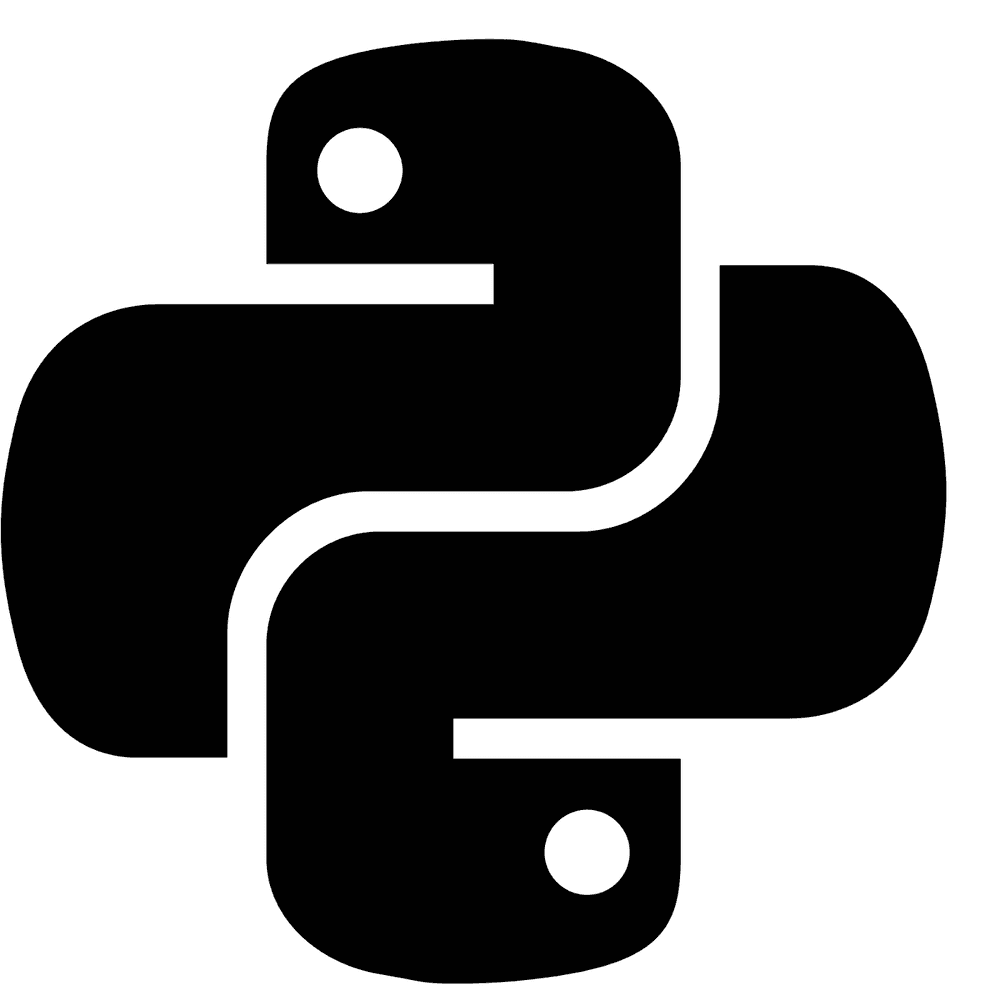# New Playground and Cheatsheet for Learning Python

29 August, 2018

I'm learning Python and I decided to create a repository where I could put Python script samples with standard Python syntax, structures and statements examples just to be able to quickly recap how to use this or that feature of the language.This is a collection of Python scripts that are split by topics and contain code examples with explanations, different use cases and links to further readings.

It is a playground because you may change or add the code to see how it works and test it out using assertions. It also allows you to lint the code you've wrote and check if it fits to Python code style guide. Altogether it might make your learning process to be more interactive and it might help you to keep code quality pretty high from very beginning.

It is a cheatsheet because you may get back to these code examples once you want to recap the syntax of standard Python statements and constructions. Also because the code is full of assertions you'll be able to see expected functions/statements output right away without launching them.

## How to Use The Repo

Each Python script in the repository has the following structure:

``````"""Lists  <--- Name of the topic here

Here might go more detailed explanation of the current topic (i.e. general info about Lists).
"""

def test_list_type():
"""Explanation of sub-topic goes here.

Each file contains test functions that illustrate sub-topics (i.e. lists type, lists methods).
"""

# Here is an example of how to build a list.  <-- Comments here explain the action
squares = [1, 4, 9, 16, 25]

# Lists can be indexed and sliced.
# Indexing returns the item.
assert squares == 1  # <-- Assertions here illustrate the result.
# Slicing returns a new list.
assert squares[-3:] == [9, 16, 25]  # <-- Assertions here illustrate the result.``````

So normally you might want to do the following:

• Find the topic you're want to learn or recap.
• Look at code examples and assertions to see usage examples and expected output.
• Change code or add new assertions to see how things work.
• Run tests and lint the code to see if it work and is written correctly.

1. Getting Started
1. Operators
• Arithmetic Operators (`+`, `-`, `*`, `/`, `//`, `%`, `**`)
• Bitwise Operators (`&`, `|`, `^`, `>>`, `<<`, `~`)
• Assignment Operators (`=`, `+=`, `-=`, `/=`, `//=` etc.)
• Comparison Operator (`==`, `!=`, `>`, `<`, `>=`, `<=`)
• Logical Operators (`and`, `or`, `not`)
• Identity Operators (`is`, `is not`)
• Membership Operators (`in`, `not in`)
1. Data Types
1. Control Flow
1. Functions
1. Classes
1. Modules
1. Errors and Exceptions
1. Files